## Algebra

Showing posts with label properties. Show all posts
Showing posts with label properties. Show all posts

### Properties of the Logarithm

The following properties of the logarithm are derived from the rules of exponents.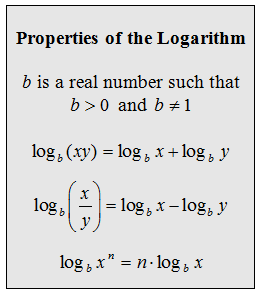The properties that follow below are derived from the fact that the logarithm is defined as the inverse of the corresponding exponential.To familiarize ourselves with the properties we will first expand the following logarithms. (Assume all variables are positive.)

Expand.Notice that there is no explicit property that allows us to work with nth roots within the argument of the logarithm.  To simplify these, first change the root to a rational exponent then apply the power rule.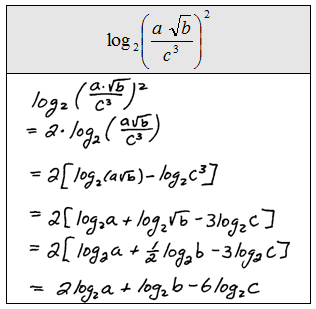When expanding, notice that we must use the same base throughout the expression. For the next set of problems we will first use the properties to expand then substitute in the appropriate values as the last step.
Evaluate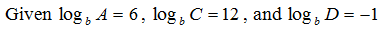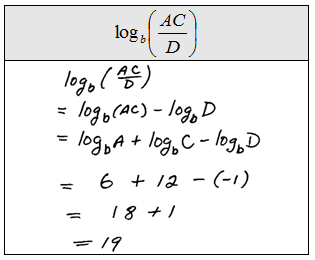Expanding is useful for learning the rules and properties associated with logarithms but as it turns out, in practice, condensing down to a single logarithm is the skill that we really need to focus on.

Rewrite as a single logarithm (condense).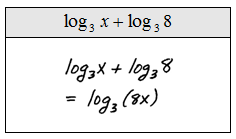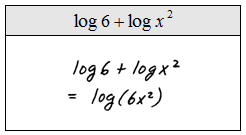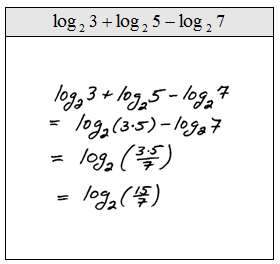Tip: When simplifying these down to one logarithm use only one operation at a time and work from left to right. Combining or skipping steps usually leads to mistakes. Do not race, work slowly, and pay attention to notation.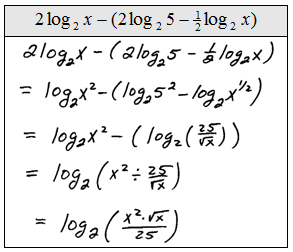Evaluate (Round to the nearest ten thousandths where appropriate).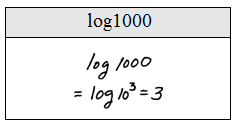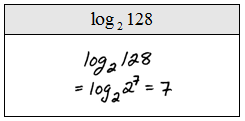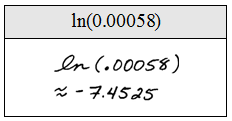Simplify.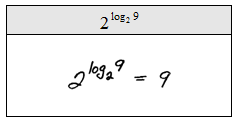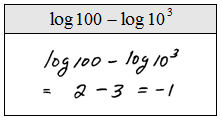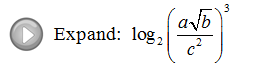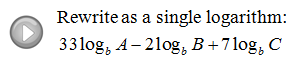### Intermediate Algebra Exam #4

Click on the 10 question exam covering topics in chapter 7. Give yourself one hour to try all of the problems and then come back and check your answers.

IA Sample Exam #4 ]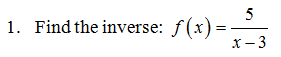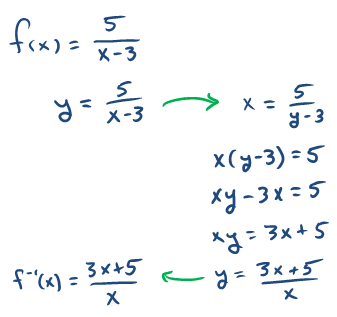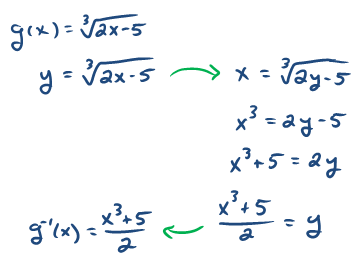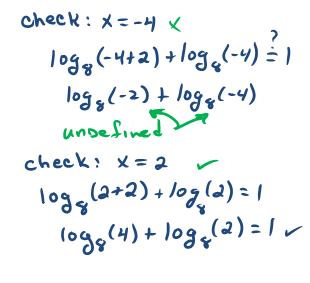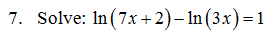8. A \$1,000 investment is made at an annual interest rate of  7 3/4% that is compounded quarterly.  How long will it take the investment to double?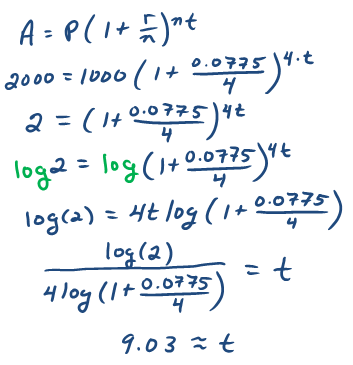9. Francium-223 has a half-life of about 22 minutes. How much of a 3-microgram sample of francium-223 will be left after 5 minutes of decay? Round off answer to the nearest hundredth of a microgram.10. In the year 2000 a certain small town had a population of 72,000 people.  In the year 2010 the population was estimated to have grown to 108,000 people. If the population continues to grow exponentially at this rate, estimate the population in the year 2016.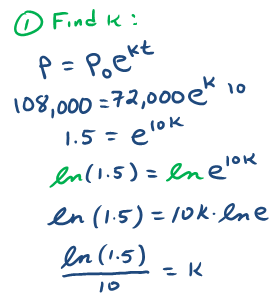---